# Lines And Angles NCERT Solutions : Class 9 Maths

## NCERT Solutions for Class 9 Maths : Lines And AnglesFilter
Filters :
Classes
•
•
•
•
•
•
•
Chapters
•
•
•
•
•
•
•
•
•
•
• 7 More
Exercises
•
•
•
•

Doubtnut one of the best online education platform provides free NCERT Solutions of Maths for Class 9 Lines And Angles which are solved by our maths experts as per the NCERT (CBSE) guidelines. We provide the solutions as video solutions in which concepts are also explained along with answer which will help students while learning , preparing for exams and in doing homework. These Solutions will help to revise complete syllabus and score more marks in examinations.

Get here free, accurate and comprehensive NCERT Solutions for Class 9 Maths Lines And Angles which have been reviewed by our maths counsellors as per the latest edition following up the CBSE guidelines. We provide video solutions in which solutions to all the questions of NCERT Class 9 Maths textbook are explained in step by step and detailed way.

## The Topics of the Chapter Lines And Angles are :

RELATION BETWEEN ANGLES

ANGLE MADE BY TRANSVERSAL WITH TWO LINES

EXTERIOR ANGLE OF A TRIANGLE

TYPES OF ANGLES

PROPERTIES OF A TRIANGLE

It contains these exercises along with solved examples. All the exercises are solved in the video. Select the exercise to view the solutions exercisewise of the Chapter Lines And Angles:

## NCERT Solutions Class 9 Maths Chapter Lines And Angles Exercises:

We have covered all the exercises and also Solved examples in the videos. Along with the practise exercise Students should also practise solved examples to clear the concepts of the Lines And Angles. If incase you have any doubt you can watch the solutions for the given questions.Watch the solutions for the given questions in which it is also explained in the video steps to solve the questions along with answers.

# NCERT Class 9 Solutions Chapter 6 LINES AND ANGLES

In the previous units, the concepts of lines and angles were covered in-depth in the NCERT book. By now, the students must have got a sound knowledge about the definitions and types of lines and angles. This chapter covers one of the important topics of properties and the relations of lines and angles. All the important concepts of this chapter are well explained in NCERT books along with plenty of examples and exercises.

NCERT books have been one of the most preferred textbooks in India by the students studying the CBSE syllabus. The students find the concepts easy to understand when learned from NCERT books, especially a subject like Maths. This is owing to the reason that NCERT books provide an easy explanation to even the difficult concepts giving various examples related to real life. The step-by-step approach followed to solve the problems are the highlights of the textbook and it makes the process of study easy for the learners.

NCERT books cover all the topics required to face the CBSE board school exams.  The NCERT books also help in cracking the other competitive entrance examinations. Class 9 marks the beginning of a crucial period that requires serious preparations from the students who want to excel in the tough entrance exams, NCERT books serve as the guiding light for them. The more you practice the more you become proficient in maths.

For a subject like Maths, it is essential that the learners practice more and more problems in order to learn the concepts of a chapter comprehensively. The NCERT textbook provides the ample number of problems that are sufficient for the students' practice. Now with a proper textbook for reference, the next important requirement for the students would be a good solution guide to the exercise questions from the textbook. The solutions guide proves to be very useful when the students try to solve the difficult textbook questions. With the help of a proper solutions guide, the students who are into self-study mode would benefit a lot.

Doubtnut provides all the solutions for the NCERT textbook in a chapter-wise manner. NCERT maths Class 9 Solutions Chapter 6 Lines and Angles are presented in the form of videos that can be accessed from anywhere and anytime. The students can rely on these solution videos as all problems, including the complex ones are broken down into simpler solutions by our experts who are available to solve students queries whenever they require.

## Lines and Angles – Class 9 Maths NCERT Solutions

In previous chapters, students have learned about lines i.e. (when a mark is formed with a pen or pencil it is called as lines) as defined earlier it can be straight, horizontal or vertical. Now further in this chapter, we will be learning about different properties of lines and angles. Let us study the current chapter in detail now.

### What are line and its parts?

We know that when a mark made with a pen or pencil it is called line and it can extend till infinity. When there are two specific points AB on line then it is called a line segment.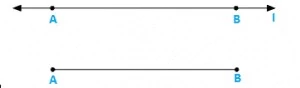Similarly, if there are three or more points lie in a single line than those points are called collinear points. Lines which do not intersect each other and are running in the opposite direction of each other are called parallel lines and when two lines intersect each other at a common point are called intersecting lines and point is called the point of intersection.

### What are angles?

When two rays originate from the same endpoint then an angle is formed between them. The rays which make the angle is called arms and the end point is called vertex of the angle. There are different types of angle on the basis of their formation: -

The angle formed between 00 and 900 is called an acute angle.  This is smaller than the right angle.

The angle which measures exactly 900 is called a right angle.

The angle which is greater than 900 and less than 1800 is called an obtuse angle.

The angle which measures 1800 is called straight angle and angle which is greater than 1800 and less then 3600 is called a reflex angle.

If the sum of two angles is 900 then they are called complementary angles, and if the sum of two angles is equal to 1800 then they are called supplementary angles.

## Topics and Subtopics of NCERT Class 9 Maths Chapter 6 – Lines and Angles

Chapter lines and angles are divided into further topics and subtopics in which the content of previous knowledge of the student is given as well as the current syllabus is covered in the form of theorems and exercises. Knowing the topics and subtopics of a given chapter helps the learners be aware of the whole content in a short form. This helps them to sort out each topic the way they want to while planning to study. Let us look at the Topics and Subtopics of NCERT Class 9 Maths Chapter 6 – Lines and angles, according to which the solutions are worked out on the website:

## Properties of lines and angles

When an angle has a common vertex (means ray) and a common point from where the rays originate then the two angles formed are called adjacent angles.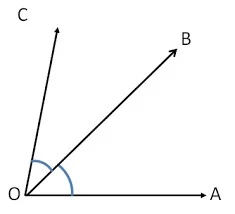In the above figure angle, COB and angle BOA are adjacent angles with a common vertex BO and common point O.

### Pair of angles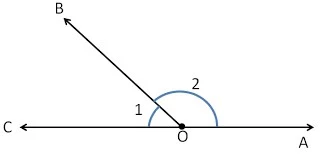A linear pair of angle is equal to 1800. Linear pair of angles are formed by two adjacent angles and are equal to 1800 i.e. straight angle. Therefore in the above figure:-

Angle COB +Angle AOB = Angle COA

Angle COB +Angle AOB = 1800

### Intersecting lines

When two lines intersect each other at a point, which is common to both lines. Then the lines are called intersecting lines and the point is called the point of intersection.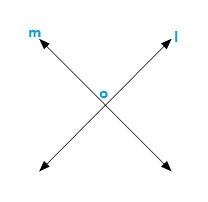Prove when two lines intersect each other, then two vertically opposite angles which are formed are equal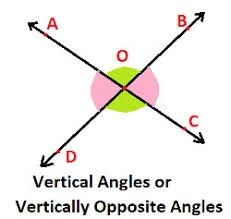With the help of this figure, we come to know that angle AOD and angle AOB is equal to 1800

And angle COD and angle COB is equal straight angle BOD which is equal to 1800

Therefore, we can write as angle AOD + angle AOB =angle AOB +angle COB

Hence, we can say that

Angle AOD = Angle BOC

Angle AOB = Angle COD

### Parallel lines

When two lines do not intersect each other at any point and are in the opposite direction of each other then they are said as parallel lines. Line l and m are examples of parallel line.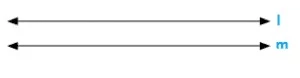### Transversal line

A line that passes through two or more parallel lines is called a transversal line and different angles are formed when the transversal line passes through the parallel lines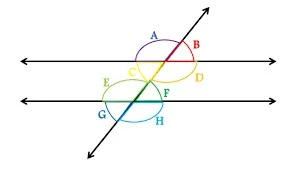Different angles formed through transversal lines are

Corresponding angles are angle A, E Angle B, F Angle C,  G, Angle D, H

Alternate Interior angles – Angle C and F, Angle D and E

Alternate Exterior angles – Angle A and H, Angle B and G

Interior angles on the same side of the transversal – Angle C and E, Angle D and F

### Angle sum property of a Triangle

According to this property, the sum of all the interior angles of the triangle is equal to 1800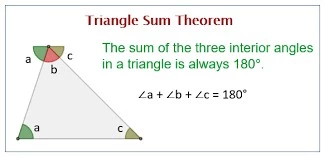Example:

Find the value of x in the following triangle.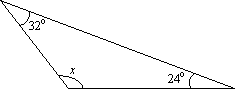Solution:

x + 24° + 32° = 180° (sum of angles is 180°)
x + 56° = 180°
x = 180° – 56° = 124°

## Significance of NCERT Mathematics Book Class 9

1. NCERT books are loaded with great study material. Ample number of exercises and examples are given of each and every topic which helps students to understand and learn the concepts in an easy and convenient language.
2. The book contains in-depth explanations of all the important concepts, which help students to understand the concepts in a better way.
3. Different methods and concepts in this book are explained in an easy to understand language that makes it quite helpful for all the students.

The students are benefitted while practicing from the NCERT book and also if they have further doubts, it can be cleared with the help of online tutorials given on Doubtnut.com, where the experts are available to help the students in every possible way. It might sound difficult in the beginning, but with the help of right study medium and materials, the students can easily master even the complex concepts. On our website, all of these concepts are taught in video format and this helps the students to learn the lessons with better concentration. The lessons taught in a step-by-step manner by an expert teacher helps in understanding the concepts in a better way. All the doubts occurring during the learning process can be easily be clarified by just posting the picture of the question on the website. Thus, Doubtnut is the right platform especially for the students who are into self-study mode. Our website makes the perfect online tutorial platform for the students facing CBSE academic exams, CBSE board exams and the other competitive entrance exams.

Share this on :-